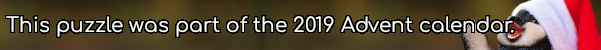mscroggs.co.uk
mscroggs.co.uksubscribe

# Puzzles

## 16 DecemberArrange the digits 1-9 in a 3×3 square so that: the median number in the first row is 6; the median number in the second row is 3; the mean of the numbers in the third row is 4; the mean of the numbers in the second column is 7; the range of the numbers in the third column is 2, The 3-digit number in the first column is today's number.
 median 6 median 3 mean 4 today's number mean 7 range 2

## Archive

Show me a random puzzle
▼ show ▼